Author: Oscar Cronquist Article last updated on July 19, 2021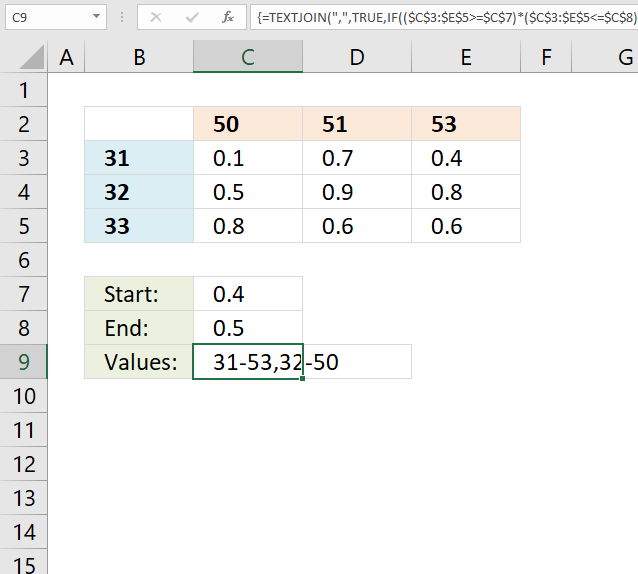This article demonstrates a few different formulas that extract values from the table column header names and the right-most column.

The image above shows the formula in cell C9, it extracts values from row two and column B if they intersect with a range of values specified in cell C7 and C8.

Example, value 0.4 is in cell E3, cells E2 and B3 are on the same column and same row respectively. The values 53 and 31 are extracted and shown in cell C9.

The Excel 2016 formula lets you concatenate all values in one cell and it works only in excel 2016 because of the TEXTJOIN function. You only need one formula and it is small and easy to understand.

The version that works with all Excel versions lets you put values in a cell each however the formulas are a little bit more complicated.

## 1. Reverse two-way lookups in a cross-reference table [Excel 2016]

I have this table where there are two dependent variables.
table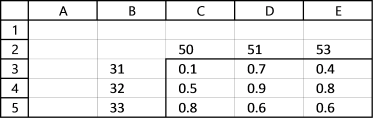Row 2 contains variable and Column B contains another variable
The combination of 32 with 51 gives me 0.9 or cell D4
I need to report which 2 and B combinations give me values between 0.4 and 0.5. In this case, it would be 31-53 and 32-50.
I could rearrange this to be in 3 columns with the results on the last column and use something like below (The equation does not represent the example I gave.)
IFERROR(INDEX(array, SMALL(IF((min=data), ROW(\$B\$2:\$B\$10)-1), ROW(A3)), COLUMN(A3)), " ")
This works but my excel table would have too many rows and the initial data set comes the other way so it would take some time to convert it. The original matrix is more compact.
I am trying to convert this equation but I am having trouble matching it to an array instead to just one column.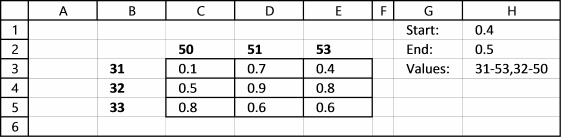The following array formula returns multiple values from a cross-reference table if they meet specific criteria.

Array formula in cell H3:

=TEXTJOIN(",", TRUE, IF((\$C\$3:\$E\$5>=\$H\$1)*(\$C\$3:\$E\$5<=\$H\$2), \$B\$3:\$B\$5&"-"&\$C\$2:\$E\$2,""))

If you prefer having the values in a cell each instead of concatenated values in one cell, go to this part of this article.

### 1.1 How to build an array formula

1. Select cell H3
2. Type the formula above in formula bar3. Press and hold CTRL + SHIFT key
4. Press Enter

If you did above steps correctly excel automatically adds a beginning and ending curly bracket to the formula, like this:Don't enter these characters yourself.

### 1.2 Explaining array formula in cell G3

Note, the TEXTJOIN function works only in Excel 2016. Use this formula if you have an earlier version of Excel.

#### Step 1 - Check which values are larger than the condition in cell H1

The less than sign and equal sign are logical operators and can be combined, the result is a boolean value True or False.

\$C\$3:\$E\$5>=\$H\$1

becomes

{0.1, 0.7, 0.4; 0.5, 0.9, 0.8; 0.8, 0.6, 0.6}>=0.4

and returns

{FALSE, TRUE, TRUE; TRUE, TRUE, TRUE; TRUE, TRUE, TRUE}.

#### Step 2 - Check which values are smaller than the condition in cell H2

\$C\$3:\$E\$5<=\$H\$2

becomes

{0.1, 0.7, 0.4; 0.5, 0.9, 0.8; 0.8, 0.6, 0.6}<=0.5

and returns

{TRUE,FALSE,TRUE; TRUE,FALSE,FALSE; FALSE,FALSE,FALSE}.

#### Step 3 - Both conditions must be TRUE (AND-logic)

(\$C\$3:\$E\$5>=\$H\$1)*(\$C\$3:\$E\$5<=\$H\$2)

becomes

{FALSE, TRUE, TRUE; TRUE, TRUE, TRUE; TRUE, TRUE, TRUE} * {TRUE,FALSE,TRUE; TRUE,FALSE,FALSE; FALSE,FALSE,FALSE}

and returns {0, 0, 1; 1, 0, 0; 0, 0, 0}.

#### Step 4 - Extract corresponding values from B3:B5 and C2:E2

The IF function returns one value if the logical test is TRUE and another value if the logical test is FALSE.

IF(logical_test, [value_if_true], [value_if_false])

IF((\$C\$3:\$E\$5>=\$H\$1)*(\$C\$3:\$E\$5<=\$H\$2), \$B\$3:\$B\$5&"-"&\$C\$2:\$E\$2,"")

becomes

IF({0,0,1;1,0,0;0,0,0}, \$B\$3:\$B\$5&"-"&\$C\$2:\$E\$2,"")

becomes

IF({0,0,1;1,0,0;0,0,0}, {"31-50","31-51","31-53";"32-50","32-51","32-53";"33-50","33-51","33-53"},"")

and returns {"","","31-53";"32-50","","";"","",""},

#### Step 5 - Concatenate values using TEXTJOIN function

The TEXTJOIN function allows you to combine text strings from multiple cell ranges and also use delimiting characters if you want.

TEXTJOIN(delimiterignore_emptytext1[text2], ...)

TEXTJOIN(",",TRUE,{"","","31-53";"32-50","","";"","",""})

returns

"31-53. 32-50" in cell H3.

Link to two-way lookups in an index table:
Looking up data in a cross reference table

#### Get excel *.xlsx file

Reverse two-way lookup in a cross reference table.xlsx

## 2. Reverse two-way lookups in a cross-reference table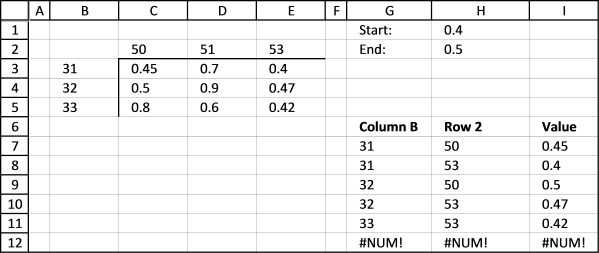This formula is for excel versions that don't have the TEXTJOIN function or if you prefer having the values in a cell each instead of concatenated values in one cell.

Array formula in cell G7:

=INDEX(\$B\$3:\$B\$5, SMALL(IF((\$C\$3:\$E\$5<=\$H\$2)*(\$C\$3:\$E\$5>=\$H\$1), MATCH(ROW(\$B\$3:\$B\$5), ROW(\$B\$3:\$B\$5)), ""), ROW(A1)))

Array formula in cell H7:

=INDEX(\$C\$2:\$E\$2, SMALL(IF((\$C\$3:\$E\$5<=\$H\$2)*(\$C\$3:\$E\$5>=\$H\$1)*(G7=\$B\$3:\$B\$5) ,MATCH(COLUMN(\$C\$2:\$E\$2), COLUMN(\$C\$2:\$E\$2)), ""), COUNTIF(\$G\$6:G7,G7)))

Array formula in cell I7:

=INDEX(\$C\$3:\$E\$5, MATCH(G7,\$B\$3:\$B\$5, 0), MATCH(H7, \$C\$2:\$E\$2, 0))

How to enter an array formula

### 2.1 Explaining formula in cell G7

#### Step 1 - Check which values are larger than the condition in cell H1

The less than sign and equal sign are logical operators and can be combined, the result is a boolean value True or False.

\$C\$3:\$E\$5>=\$H\$1

becomes

{0.45, 0.7, 0.4; 0.5, 0.9, 0.47; 0.8, 0.6, 0.42}>=0.4

and returns

{TRUE, TRUE, TRUE;TRUE, TRUE, TRUE;TRUE, TRUE, TRUE}.

#### Step 2 - Check which values are smaller than the condition in cell H2

\$C\$3:\$E\$5<=\$H\$2

becomes

{0.45, 0.7, 0.4; 0.5, 0.9, 0.47; 0.8, 0.6, 0.42}<=0.5

and returns

{TRUE, FALSE, TRUE; TRUE, FALSE, TRUE; FALSE, FALSE, TRUE}.

#### Step 3 - Both conditions must be TRUE (AND-logic)

(\$C\$3:\$E\$5>=\$H\$1)*(\$C\$3:\$E\$5<=\$H\$2)

becomes

{TRUE, TRUE, TRUE;TRUE, TRUE, TRUE;TRUE, TRUE, TRUE} * {TRUE, FALSE, TRUE; TRUE, FALSE, TRUE; FALSE, FALSE, TRUE}

and returns {1, 0, 1;1, 0, 1;0, 0, 1}.

#### Step 4 - Create a sequence from 1 to n

The ROW function returns row numbers based on a cell reference.

ROW(\$B\$3:\$B\$5)

returns {3; 4; 5}.

The MATCH function returns the relative position of an item in an array or cell reference that matches a specified value in a specific order.

MATCH(lookup_value, lookup_array, [match_type])

MATCH(ROW(\$B\$3:\$B\$5), ROW(\$B\$3:\$B\$5))

becomes

MATCH({3; 4; 5}, {3; 4; 5})

and returns {1; 2; 3}.

#### Step 5 - Return corresponding row number if True

The IF function returns one value if the logical test is TRUE and another value if the logical test is FALSE.

IF(logical_test, [value_if_true], [value_if_false])

IF((\$C\$3:\$E\$5<=\$H\$2)*(\$C\$3:\$E\$5>=\$H\$1)*(G7=\$B\$3:\$B\$5) ,MATCH(COLUMN(\$C\$2:\$E\$2), COLUMN(\$C\$2:\$E\$2)), "")

becomes

IF({1, 0, 1;1, 0, 1;0, 0, 1}, {1; 2; 3}, "")

and returns {1,"", 1; 2, "", 2; "", "", 3}.

#### Step 6 - Extract k-th smallest row number

The SMALL function returns the k-th smallest value from a group of numbers.

SMALL(arrayk)

SMALL(IF((\$C\$3:\$E\$5<=\$H\$2)*(\$C\$3:\$E\$5>=\$H\$1), MATCH(ROW(\$B\$3:\$B\$5), ROW(\$B\$3:\$B\$5)), ""), ROW(A1))

becomes

SMALL({1,"", 1; 2, "", 2; "", "", 3}, ROW(A1))

becomes

SMALL({1,"", 1; 2, "", 2; "", "", 3}, 1)

and returns 1.

#### Step 7 - Return value based on a row number

The INDEX function returns a value from a cell range, you specify which value based on a row and column number.

INDEX(array, [row_num], [column_num])

INDEX(\$B\$3:\$B\$5, SMALL(IF((\$C\$3:\$E\$5<=\$H\$2)*(\$C\$3:\$E\$5>=\$H\$1), MATCH(ROW(\$B\$3:\$B\$5), ROW(\$B\$3:\$B\$5)), ""), ROW(A1)))

becomes

INDEX(\$B\$3:\$B\$5, 1)

amd returns 31.

### 2.3 Explaining formula in cell I7

#### Get excel *.xlsx file

Reverse-two-way-lookup-in-a-cross-reference-tablev3.xlsx

## 3. Reverse two-way lookup in a cross-reference table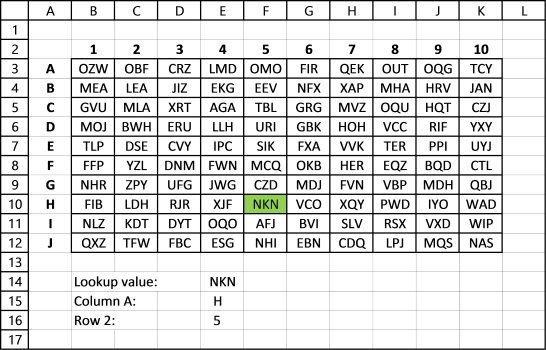The following array formulas return a single value from a cross-reference table.

Array formula in cell E15:

=INDEX(\$A\$3:\$A\$12, MIN(IF(B3:K12=E14, MATCH(ROW(B3:K12), ROW(B3:K12)), "")))

Array formula in cell E16:

=INDEX(\$B\$2:\$K\$2, MIN(IF(B3:K12=E14, MATCH(COLUMN(B3:K12), COLUMN(B3:K12)), "")))

How to enter an array formula

### 3.1 Explaining array formula in cell E15

#### Step 1 - Check what values in cell range equals the value in E14 and return the smallest row

MIN(IF(B3:K12=E14, MATCH(ROW(B3:K12), ROW(B3:K12)), ""))

returns 5.

#### Step 2 - Return corresponding header value

=INDEX(\$A\$3:\$A\$12, 5)

returns H.

### Get the Excel fileReverse-two-way-lookup-in-a-cross-reference-tablev2.xlsx